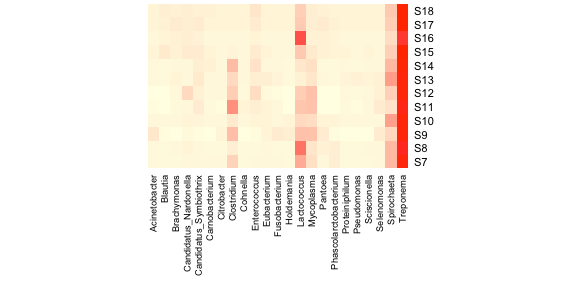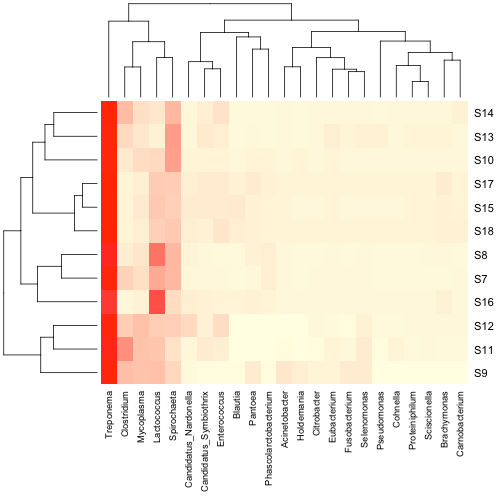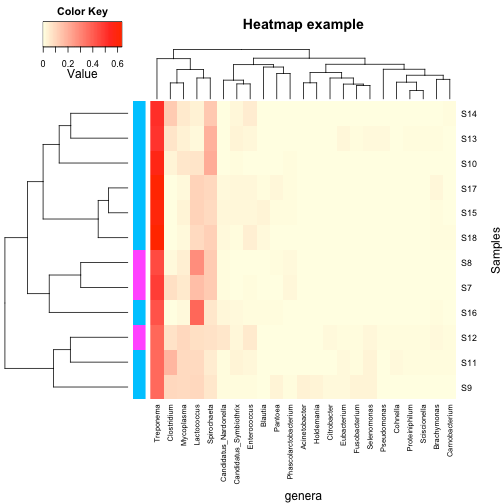# Making heatmaps with R for microbiome analysisArianne Albert is the Biostatistician for the Women’s Health Research Institute at the British Columbia Women’s Hospital and Health Centre. She earned a PhD from the University of British Columbia under the tutelage of Dolph Schluter before branching off into health research. Arianne currently has her fingers in all kinds of research pies, including work on the vaginal microbiome. She still has a soft spot for sticklebacks though. You can find her on LinkedIn and Google Scholar.

Heatmaps are incredibly useful for the visual display of microarray data or data from high-trhoughput sequencing studies such as microbiome analysis. Basically, they are false colour images where cells in the matrix with high relative values are coloured differently from those with low relative values. Heatmaps can range from very simple blocks of colour with lists along 2 sides, or they can include information about hierarchical clustering, and/or values of other covariates of interest. Fortunately, R provides lots of options for constructing and annotating heatmaps.

### R preliminaries

The first step is to make sure you’ve got the right libraries loaded. I find that the heatmap function in the basic stats package (loaded by default) is quite useful for many applications. However, more complicated annotations require either gplots or Heatplus (from Bioconductor).

`library(gplots)  # for heatmap.2`

```# to install packages from Bioconductor:
source("http://bioconductor.org/biocLite.R")
biocLite("Heatplus")  # annHeatmap or annHeatmap2
library(Heatplus)```

```# load the vegan package for hierachical clustering if you want to use distance functions not specified in dist.
library(vegan)```

```# load the RColorBrewer package for better colour options
library(RColorBrewer)
```

#### Get and Prepare Dataset

Next, we need some data! For this example, I’m going to use some microbiome data available for download from Dryad, originally from the paper:

Boucias DG, Cai Y, Sun Y, Lietze V, Sen R, Raychoudhury R, Scharf ME. 2013. The hindgut-lumen prokaryotic microbiota of the lignocellulose-degrading termite Reticulitermes flavipes and its responses to dietary lignocellulose composition. Molecular Ecology 22(7): 1836–1853. doi:10.1111/mec.12230; Dryad data package doi:10.5061/dryad.b922k.

This file is not formatted in a way that R will understand, so it requires some alteration before importing. The file has samples across the top and genera along the rows as well as some lovely, but superfluous, summary annotation. I got rid of extra rows and columns and transposed the rest in Excel. Now the 238 genera are along the top with the 12 samples as the rows.

Load your data! I’m assuuming you know how to do this in R …

```all.data <- read.csv("genera example.csv")  # load the data
dim(all.data)
  12 239
```

Here are the first 3 rows and 4 columns to give you an idea of what the data should look like:

```all.data[1:3, 1:4]
sample Acetivibrio Acetobacter Achromobacter
1     S7           0           5             0
2     S8           1           0             1
3     S9           0           3             1
```

We’ll have to strip off the sample ids and convert them to row names so that the data matrix contains only sequence count data.

```row.names(all.data) <- all.data\$sample
all.data <- all.data[, -1]
```

Now, to make a heatmap with microbiome sequencing data, we ought to first transform the raw counts of reads to proportions within a sample:

```data.prop <- all.data/rowSums(all.data)
data.prop[1:3, 1:3]
Acetivibrio Acetobacter Achromobacter
S7   0.000e+00   0.0004289     0.000e+00
S8   9.625e-05   0.0000000     9.625e-05
S9   0.000e+00   0.0002759     9.195e-05
```

### Make heatmaps

Generally, the default colours for heatmaps are pretty appalling. I personally like this palette, but play around to see what suits you.

```# colorRampPalette is in the RColorBrewer package.  This creates a colour palette that shades from light yellow to red in RGB space with 100 unique colours
scaleyellowred <- colorRampPalette(c("lightyellow", "red"), space = "rgb")(100)```

The most basic of heatmaps:

```heatmap(as.matrix(data.prop), Rowv = NA, Colv = NA, col = scaleyellowred)
```It’s pretty clear that this plot is inadequate in many ways. For one, the genus labels are all squished along the bottom and impossible to read. One solution to this problem is to remove genera that are exceedingly rare from this figure. Let’s try removing genera whose relative read abundance is less than 1% of at least 1 sample.

```# determine the maximum relative abundance for each column
maxab <- apply(data.prop, 2, max)
Acetivibrio     Acetobacter   Achromobacter Acidaminococcus
1.088e-04       5.972e-04       5.441e-04       8.578e-05
```

```# remove the genera with less than 1% as their maximum relative abundance
n1 <- names(which(maxab < 0.01))
data.prop.1 <- data.prop[, -which(names(data.prop) %in% n1)]
```

This leaves us with 23 genera suggesting that most of the taxa sampled occur at very low relative abundances. The heatmap with the new data looks like this, with a bit of extra fiddling to get all of the labels displayed.

```# the margins command sets the width of the white space around the plot. The first element is the bottom margin and the second is the right margin
heatmap(as.matrix(data.prop.1), Rowv = NA, Colv = NA, col = scaleyellowred, margins = c(10, 2))
```Much better! Now let’s add a dendrogram for the samples. The heatmap function will do this for you, but I prefer to make my own using the vegan package as it has more options for distance metrics. Also, this means that you can do hierarchical clustering using the full dataset, but only display the more abundant taxa in the heatmap.

```# calculate the Bray-Curtis dissimilarity matrix on the full dataset:
data.dist <- vegdist(data.prop, method = "bray")```

```# Do average linkage hierarchical clustering. Other options are 'complete' or 'single'. You'll need to choose the one that best fits the needs of your situation and your data.
row.clus <- hclust(data.dist, "aver")```

```# make the heatmap with Rowv = as.dendrogram(row.clus)
heatmap(as.matrix(data.prop.1), Rowv = as.dendrogram(row.clus), Colv = NA, col = scaleyellowred, margins = c(10, 3))
```You can also add a column dendrogram to cluster the genera that occur more often together. Note that this one must be done on the same dataset that is used in the Heatmap (i.e. reduced number of genera).

```# you have to transpose the dataset to get the genera as rows
data.dist.g <- vegdist(t(data.prop.1), method = "bray")
col.clus <- hclust(data.dist.g, "aver")```

```# make the heatmap with Rowv = as.dendrogram(row.clus)
heatmap(as.matrix(data.prop.1), Rowv = as.dendrogram(row.clus), Colv = as.dendrogram(col.clus), col = scaleyellowred, margins = c(10, 3))
```For some applications, the above heatmaps would be sufficient, however, there are many times where it would be nice to indicate on the heatmap values for other important variables, such as experimental groups, or values of covariates. Also, it would be nice to have a legend for the colour scale. heatmap.2 in the gplots package can do both of these things.

First, let’s make up some annotation for our dataset (I would like to be 100% clear that this is totally fictional data and not in the original publication in any way):

```# There are 12 samples in the dataset, so let's assign them randomly to one of two fictional categories
var1 <- round(runif(n = 12, min = 1, max = 2))  # this randomly samples from a uniform distribution and rounds the result to an integer value```

```# change the 1s and 2s to the names of colours:
var1 <- replace(var1, which(var1 == 1), "deepskyblue")
var1 <- replace(var1, which(var1 == 2), "magenta")```

```# if this was real data, you would need the order of the values to be in the same order as the sequencing data e.g.
cbind(row.names(data.prop), var1)
```

```var1
[1,] S7  magenta
[2,] S8  magenta
[3,] S9  deepskyblue
[4,] S10 deepskyblue
[5,] S11 deepskyblue
[6,] S12 magenta
[7,] S13 deepskyblue
[8,] S14 deepskyblue
[9,] S15 deepskyblue
[10,] S16 deepskyblue
[11,] S17 deepskyblue
[12,] S18 deepskyblue
```

Next, we can make a more complicated heatmap.

```heatmap.2(as.matrix(data.prop.1),Rowv = as.dendrogram(row.clus), Colv = as.dendrogram(col.clus), col = scaleyellowred, RowSideColors = var1, # this puts in the annotation for the samples margins = c(10, 3))
```You’ll note that some of the defaults for heatmap.2 are kind of funny looking. The green tracing in each column can be turned off with `trace = "none"`, and the histogram in the scale legend can be removed with `density.info = "none"`. You can add plot labels (e.g. `main`, `xlab` and `ylab`), as well as change the relative sizing of the plot elements (`lhei` and `lwid`). There are lots of other options which are surprisingly well described in the help for `heatmap.2`.

```heatmap.2(as.matrix(data.prop.1), Rowv = as.dendrogram(row.clus), Colv = as.dendrogram(col.clus), col = scaleyellowred, RowSideColors = var1, margins = c(11, 5), trace = "none", density.info = "none", xlab = "genera", ylab = "Samples", main = "Heatmap example", lhei = c(2, 8)) # this makes the colour-key legend a little thinner
```Finally, the least intuitive function is annHeatmap2 from the Heatplus package. However, it allows for annotation with multiple variables at once, as well as an option for colouring the dendrogram into clusters. All of the options within the function are written as lists, which can take some getting used to if you don’t use them regularly.

```# the annHeatmap2 function needs to be wrapped in the plot function in order to display the results
plot(annHeatmap2(as.matrix(data.prop.1),```

```# the colours have to be fiddled with a little more than with the other functions. With 50 breaks in the heatmap densities, there needs to be 51 colours in the scale palette. Error messages from the plotting should help to determine how many colours are needed for a given number of breaks
col = colorRampPalette(c("lightyellow", "red"), space = "rgb")(51), breaks = 50,```

```# dendrogram controls how dendrograms are made and can be calculatated witin the function. The internal calculation can be overridden and externally calculated dendrograms can be displayed.
dendrogram = list(Row = list(dendro = as.dendrogram(row.clus)), Col = list(dendro = as.dendrogram(col.clus))), legend = 3, # this puts the colour-scale legend on the plot. The number indicates the side on which to plot it (1 = bottom, 2 = left, 3 = top, 4 = right)
labels = list(Col = list(nrow = 12)) # gives more space for the Genus names
))
```Annotations are added with the ann sublist which takes a data frame as its input. Unlike the other functions, it can take multiple variables for annotation including continuous variables.

```# make a data frame of variables for annotation
ann.dat <- data.frame(var1 = c(rep("cat1", 4), rep("cat2", 8)), var2 = rnorm(12,  mean = 50, sd = 20))
```

```plot(annHeatmap2(as.matrix(data.prop.1), col = colorRampPalette(c("lightyellow", "red"), space = "rgb")(51), breaks = 50, dendrogram = list(Row = list(dendro = as.dendrogram(row.clus)), Col = list(dendro = as.dendrogram(col.clus))), legend = 3, labels = list(Col = list(nrow = 12)), ann = list(Row = list(data = ann.dat))))
```Finally, clusters in the dedrogram(s) can be highlighted using different colours with the cluster sublist:

```# make a data frame of variables for annotation
ann.dat <- data.frame(var1 = c(rep("cat1", 4), rep("cat2", 8)), var2 = rnorm(12, mean = 50, sd = 20))```

```plot(annHeatmap2(as.matrix(data.prop.1),
col = colorRampPalette(c("lightyellow", "red"), space = "rgb")(51),
breaks = 50,
dendrogram = list(Row = list(dendro = as.dendrogram(row.clus)), Col = list(dendro = as.dendrogram(col.clus))),
legend = 3,
labels = list(Col = list(nrow = 12)),
ann = list(Row = list(data = ann.dat)),
cluster = list(Row = list(cuth = 0.25, col = brewer.pal(3, "Set2"))) # cuth gives the height at which the dedrogram should be cut to form clusters, and col specifies the colours for the clusters
))
```This is just a taste of what these functions can do. Experimenting with changing the default values and seeing what happens is half the fun with R.